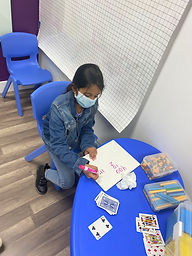Ms. Briana

Target 1​

Lesson Type:

New

Number Operation

:

Integer Composition

Understand that the digits in a four-digit number represent the amounts of thousands, hundreds, tens, and ones.

1:

Represent the composition or decomposition of three-digit numbers with an addition equation (113 = 100+10+3 or 1 hundred 1 ten and 3 ones).

2:

Understand how to “regroup” based on place-value understandings (i.e., ten ones can be composed into one ten – ten tens can be composed into one hundred).

3:

Represent the composition or decomposition of three-digit numbers with a standard expanded format equation using both multiplication and addition (384 = (3x100) +(8x10) +(4x1)).

2nd

Vocabulary:

Expanded form: Breaking a quantity down into its different place values

Activities:

Students drew cards from a deck and used them to create quantities to represent in expanded form.

Students used expanded form to solve addition and subtraction equations.Home Exploration

Guiding Questions:Absent Students:

Target 2

:

1:

Understand that the previous place (one place smaller) is the key digit to evaluate to determine whether a number is rounded up or down.

2:

Understand that rounding identifies the multiple of 10 or 100 that is closest to the given number.

3:

Use understanding of how to round two-digit numbers and expand the strategy to three-digit numbers.

3rd

Vocabulary:

Rounding means replacing a number with an approximate value that has a shorter, simpler, or more explicit representation.

Activities:

Students practiced using playing cards to create numbers to round to the nearest 10.

Students played a rounding board game using dice and rounding the number the dice created.Home Exploration

Guiding Questions:Target 3

:

Vocabulary:

Activities:Home Exploration